# Korovkin-type approximation theory

Originating from Korovkin's theorems (cf. Korovkin theorems), this theory consists of a collection of results whose main objective is to investigate under what circumstances the convergence of a (particular) sequence (more generally, net) of linear operators acting on a topological vector space is, in fact, a consequence of its convergence on special (possibly finite) subsets.

More precisely, letandbe topological vector spaces, and consider classesand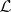of continuous linear operators fromto(cf. also Linear operator; Topological vector space).

For a linear operator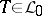, a subset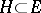is called a Korovkin subset of(forwith respect to) if for every equicontinuous net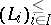(cf. also Equicontinuity) of operators insatisfying(a1)

one has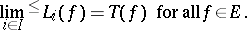(a2)

One of the main problems in this theory is to state sufficient (and necessary) conditions under which a given subset is a Korovkin subset in(forwith respect to). Such theorems are called Korovkin-type theorems.

Similar problems have been settled for netsof operators that are not necessarily equicontinuous or linear.

Another important aspect of the theory is the characterization of the Korovkin closure, or Korovkin shadow, of a given subset(forwith respect to), which is defined as the subspace of all elements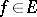such that ifis an equicontinuous net of operators insatisfying (a1), then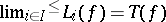.

Korovkin-type approximation theory has been developed in the context of classical function spaces and in more abstract spaces, such as locally convex ordered spaces, Banach lattices, Banach algebras, Banach spaces, etc.

Powerful and fruitful connections of this theory have been discovered not only with classical approximation theory, but also with fields such as: functional analysis; harmonic analysis; measure theory; probability theory; potential theory; and the theory of partial differential equations (cf. Differential equation, partial).